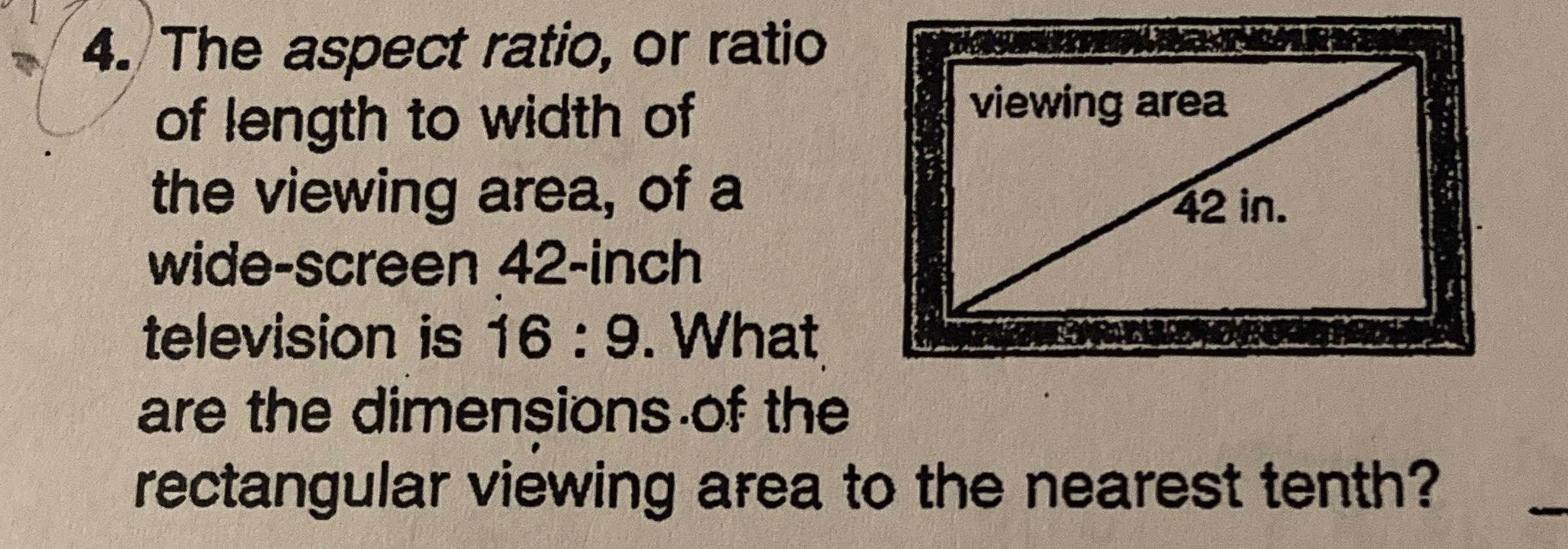### ¿Todavía tienes preguntas de matemáticas?

Pregunte a nuestros tutores expertos
Algebra
Pregunta4. The aspect ratio, or ratio of length to width of the viewing area, of a wide-screen $$42$$ -inch television is $$16 : 9$$ . What are the dimensions of the rectangular viewing area to the nearest tenth?

$$16: 9: \sqrt{337}= l: w: 42\\\Rightarrow l= 36.6,w= 20.6$$